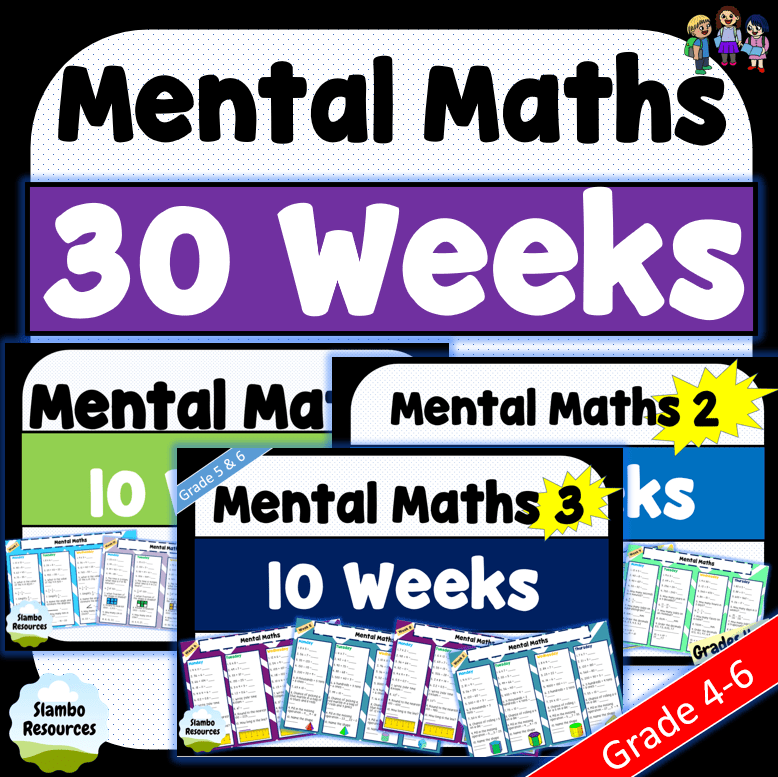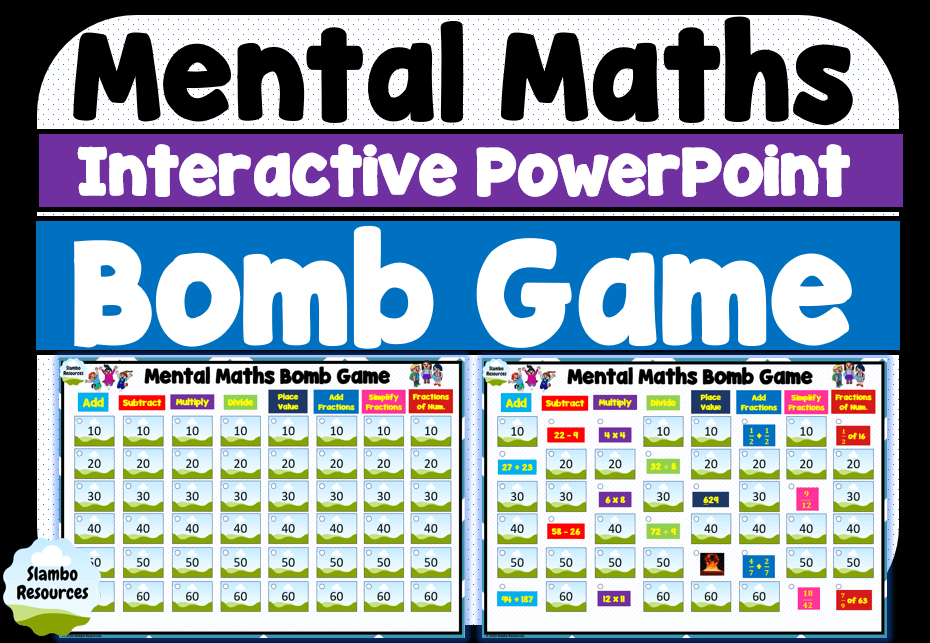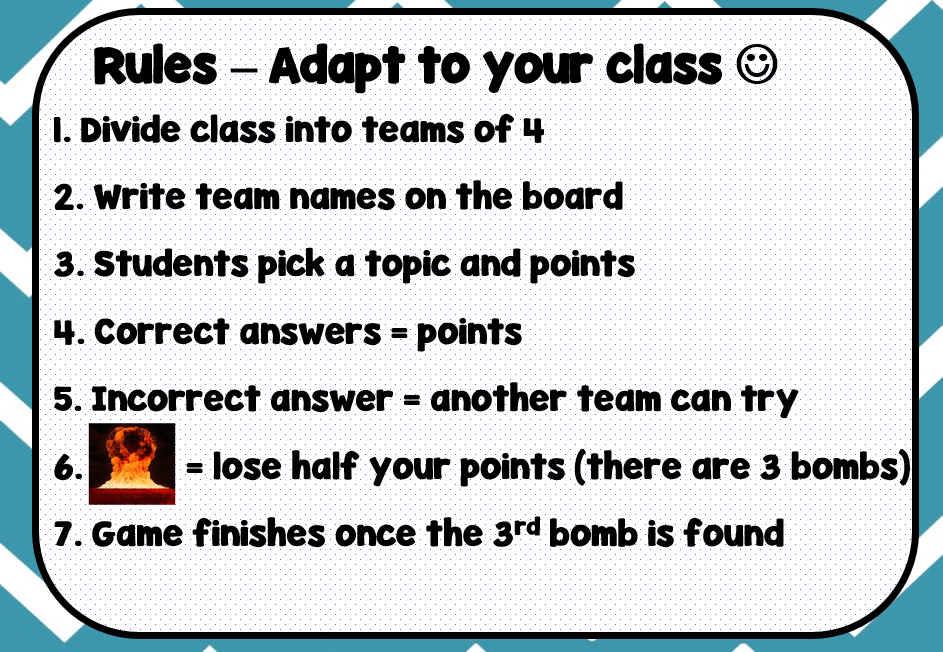## Grade 5 Mental Math Worksheets

Free grade 5 mental math worksheets to help your students develop their efficiency in Mathematics. Doing daily mental math tasks is important for students to improve their speed in maths and consolidate what they have learned. These free worksheets will help your students get the repetition they need. They cover concepts such as multiplying by 10s, dividing large numbers, addition, subtraction, area, adding & subtraction fractions, indices, conversions, ordering decimals and algebra. Use the worksheets in your daily math warm up or give it to your students for homework. Either way, they will get the practice they need and improve in no time at all! If these are too easy or too challenging, check out grade 4 mental math worksheets or grade 6 mental math worksheets! Also, check out the division mental math strategy down below! For loads and loads of mental math worksheets, have a look around my TpT store by clicking on the images below.

## Multiplication & Division Mental Math

Click on the blue button and “purchase” them for free! You don’t need to enter any credit card details, simply click purchase on the next page and your download will appear.30 weeks of daily mental maths. This bundle includes all 3 Grade 4/5 Mental Maths in this series.## Mental Math Strategy - Division

Completing division questions quickly and accurately is important. This mental math strategy can help your students improve their efficiency and provides another strategy for them to draw upon.

In my class, I teach my students to use multiplication when dividing. Instead of dividing the number from left to right, work from right to left and multiply.

Example 1: 36 ÷ 9 = ___ Work from right to left. 9 x ___ = 36. The answer is 4.

Example 2: 48 ÷ 8 = ___ Work from right to left. 8 x ___ = 48. The answer is 6.

Example 3: 63 ÷ 7 = ___ Work from right to left. 7 x ___ = 63. The answer is 9.

It’s a simple strategy that some students do naturally. If you teach this explicitly to your class and practice daily, you’ll see their progress in no time! The mental math worksheets above will provide your students the practice they need when working on this division strategy! If you need more mental math strategies, check out Third Space Learning. Their blog on mental math breaks down the importance of mental math strategies and provides a range of techniques for your students use.